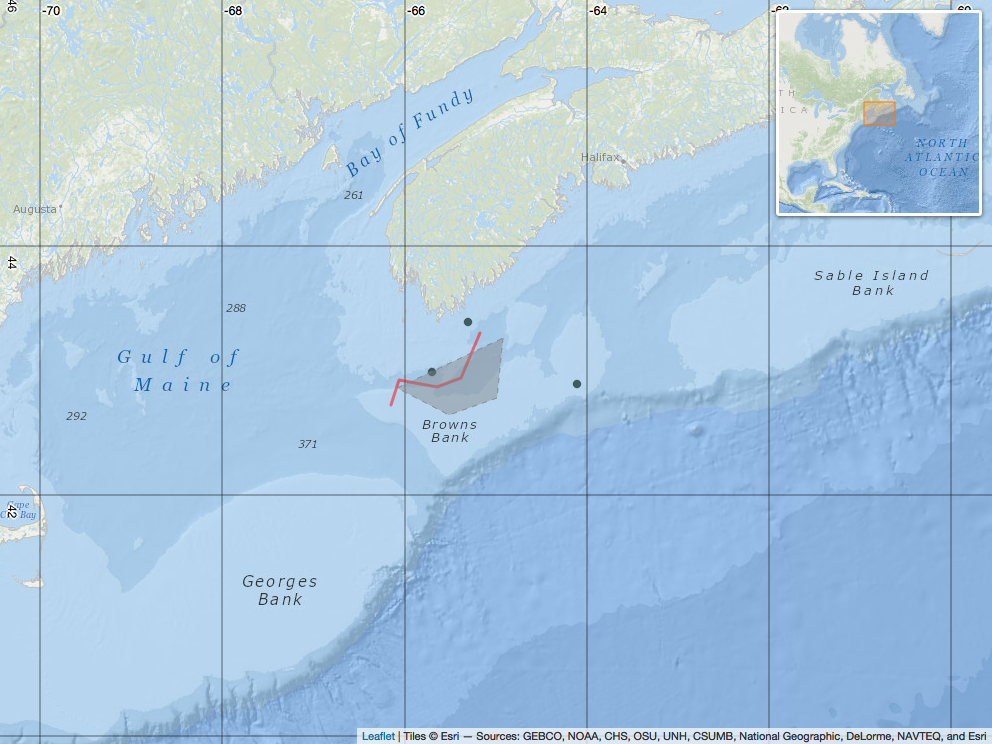# Leaflet map with inset in R

I was recently asked to expand on my post on making interactive maps to show how to produce a leaflet map with an inset map and nice lat/lon labels of suitable quality to be used in publication. Here’s a very quick example of how that might be done. The astute will notice many similarities with my last post on mapping with leaflet.

## Step 1: make some data

Here’s some test data to plot. These structures could, of course, be replaced by real data.

``````# make a simple track line
lin = data.frame(lon = c(-65.17536, -65.37423, -65.64541, -66.06122, -66.15161),
lat = c(43.30837, 42.94679, 42.87448, 42.92871, 42.72985))

# make a few points
pts = data.frame(lon = c(-65.3, -65.7, -64.1),
lat = c(43.4, 43, 42.9))

# build a polygon (in this case the 'Roseway Basin Area To Be Avoided')
ply = data.frame(lon = c(-64.916667, -64.983333, -65.516667, -66.083333),
lat = c(43.266667, 42.783333, 42.65, 42.866667))``````

## Step 2: build map

Now let’s build the map. This is achieved with the `leaflet()` function, and a series of pipes (`%>%`) to sequentially add more features. Hopefully the comments adequately describe each feature. The whole thing is stored as the variable `map`.

``````# required libraries
library(leaflet, quietly = T, warn.conflicts = F)
library(mapview, quietly = T, warn.conflicts = F)

# start basemap (note the argument to hide the zoom buttons)
map <- leaflet(options = leafletOptions(zoomControl = FALSE)) %>%

# focus map in a certain area / zoom level
setView(lng = -65, lat = 43, zoom = 7) %>%

tiles = providers\$Esri.OceanBasemap,
position = 'topright',
width = 200, height = 200,
toggleDisplay = FALSE) %>%

# add graticules with nice labels (recommended for static plot)

# add graticules from a NOAA webserver (recommended for interactive plot)
#   "https://gis.ngdc.noaa.gov/arcgis/services/graticule/MapServer/WMSServer/",
#   layers = c("1-degree grid", "5-degree grid"),
#   options = WMSTileOptions(format = "image/png8", transparent = TRUE),
#   attribution = NULL,group = 'Graticules') %>%

# add points (as circle markers)
weight = 0.5,
col = 'black',
fillColor = 'darkslategrey',
fillOpacity = 0.9,
stroke = T,
label = ~paste0('Point at: ',
as.character(round(lat,3)), ', ',
as.character(round(lon,3))),
group = 'Points') %>%

weight = 3,
color = 'red',
popup = 'This is a line!',
smoothFactor = 3,
group = 'Lines') %>%

weight = 1,
color = 'grey',
fillColor = 'grey',
fill = T,
fillOpacity = 0.25,
stroke = T,
dashArray = c(5,5),
smoothFactor = 3,
options = pathOptions(clickable = F),
group = 'Polygons')

# show map
# map

# save map as static image
# mapshot(map, file = 'leaflet_map.png')``````

Here’s the saved map: